×#### Thank you for registering.

One of our academic counsellors will contact you within 1 working day.

Click to Chat

1800-1023-196

+91-120-4616500

CART 0

• 0

MY CART (5)

Use Coupon: CART20 and get 20% off on all online Study Material

ITEM
DETAILS
MRP
DISCOUNT
FINAL PRICE
Total Price: Rs.

There are no items in this cart.
Continue Shopping```Mean Value Theorem

Table of Content

History of Mean Value Theorem

What is Mean Value Theorem?

Graphical Interpretation of Mean Value Theorem

Proof of the Mean Value Theorem

What is Rolle’s Theorem in calculus?

Graphical representation of the Rolle’s Theorem

What is the difference between the mean value theorem and the Rolle’s Theorem?

History of Mean Value Theorem

Mean Value Theorem was first defined by Vatasseri Parameshvara Nambudiri (a famous Indian mathematician and astronomer), from the Kerala school of astronomy and mathematics in India in the modern form, it was proved by Cauchy in 1823.

Its special form of theorem was proved by Michel Rolle in 1691; hence it was named as Rolle’s Theorem. It was Moritz Wilhelm Drobisch of Germany who used this name first in 1834 and then Giusto Bellavitis of Italy in 1846.

What is Mean Value Theorem?

The Mean Value Theorem states that for any given curve between two endpoints, there must be a point at which the slope of the tangent to the curve is same as the slope of the secant through its endpoints.

If f(x) is a function, so that f(x) is continuous on the close interval [a, b] and also differentiable on the open interval (a, b), then there is point c in (a, b) that is, a < c < b such that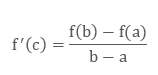The mean value theorem is also known as Lagrange’s Mean Value Theorem or first mean value theorem.

Graphical Interpretation of Mean Value Theorem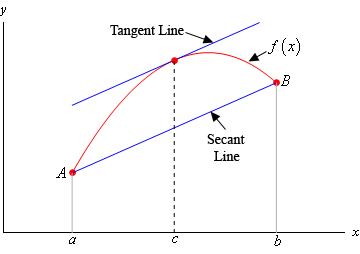Here the above figure shows the graph of function f(x).

Let A = (a, f (a)) and B = (b, f (b))

At point c where the tangent passes through the curve is (c, f(c)).

The slope of the tangent line is same as secant line that is, both are parallel to each other.

Proof of the Mean Value Theorem

To prove the expression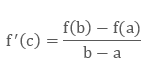We have to assume

h(x) = f(x) –sx                (i)

Where s is a constant.

As we know that function is continuous on [a, b] and differentiable on (a, b), so the function h(x) is also having the same properties.

Now according to Rolle’s TheoremBy Rolle's Theorem, as function ‘h’ is {\displaystyle g}differentiable and {\displaystyle g(a)=g(b)}h (a) = h (b) so that hꞌ(c) = 0.

Hence h(c) = f(c) –sx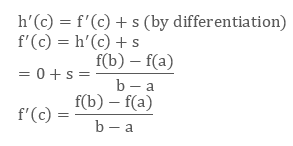Example

If a, b, are two numbers with a < b, show that a real number c can be found between a and b such the 3c2 = b2 + ab + a2.

Solution: Consider the function f(x) = x3

It is continuous and differentiable in (a, b).

Hence by LMVT, there exist a point c such that  a < c < b and f’(c) = f'(c) = f(b)–f(a)/b–a

f'(x) = 3x2, we get 3c2 = b3–a3/b–a = b2 + ba + a2.

What is Rolle’s Theorem in calculus?

It is a special case of mean value theorem which says that if y = f (x) be a given function and satisfies the conditions:

(i) f (x) is continuous on the closed interval [a , b]

(ii) f (x) is differentiable on the open interval (a , b )

(iii) f (a) = f (b)

Then there must be one point c which comes between a and b so that a < c < b.

Hence f'(c) = 0 at least once for some x ∈ (a, b)

that is,  the first derivative is zero or you can say that the slope of the tangent line to the curve of function is zero.

Note:

(1) There can be more than one such c.

(2) Think! The conditions of Rolle’s theorem are necessary or sufficient or both? The answer is conditions are only sufficient and necessary will be clear from the following examples:

(a) Let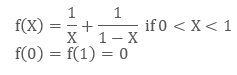Here condition (i) is violated

However f ‘(x) = 0 if x = 1/2 ∈ (0, 1)

By defining f(0) = 0 and f(1) = 3 we can see that the result is true when (i) and (iii) are violated.

(b) Let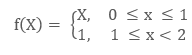Clearly (ii) does not hold in (0, 2) and even so f'(3/2) = 0

(3) If f(x) is any polynomial then between any pair of roots of f (x) = 0 lies a root of f ’(x) = 0

Graphical representation of the Rolle’s Theorem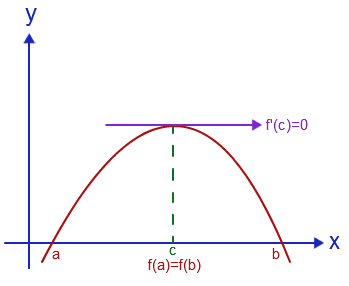Here the graph of function y = f(x) is continuous between x = a and x = b. there could be so many tangents but at least one tangent is there which is parallel to x axis. At that point fꞌ(c) = 0.

Example 1:

If ax2 + bx + c = 0, a, b, c ∈ R. Find the condition that this equation would have at least one root in (0, 1).

Solution: Let f’(x) = ax2 + bx + c

Integrating both sides,

=> f(x) = ax3 / 3 + bx2 / 2 + cx + d

=> f(0) = d and f(1) = a/3 + b/2 + c + d

Since, Rolle’s theorem is applicable

=> f(0) = f(1) => d = a/3 + b/2 + c + d

=> 2a + 3b + 6c = 0

Hence required condition is 2a + 3b + 6c = 0

Example 2:

Find the function is constant function or not? f(x) = 1/x at a = -1 and b = 1.

Solution: For function f(x) = 1/x where a = -1 and b = 1.

We have to solve the right hand side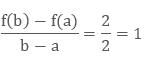On the left hand, for any cε (-1,1), ∈ not equal to 0, we have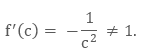So the equation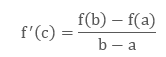does not have a solution in c. This does not contradict the Mean Value Theorem, since f(x) is not even continuous on [-1, 1].

This shows that the first derivative of a constant function is 0.

Let f(x) be a differentiable function on an interval I, with f '(x) = 0, for every x ϵ I. Then for any a and b in I, the Mean Value Theorem impliesThere is some ‘c’ between a and b. So we implies our assumption

f(b) – f(a) = 0. (b – a) = 0.

Thus f (b) = f (a) for any a and b in I, which means that f(x) is constant.

Example 3:

Show that the equation 2x3 + 3x2 + 6x + 1 = 0 has exactly one real root.

Solution: Let the function as f(x) = 2x3 + 3x2 + 6x + 1.

Now we have

f (0) = 2x3 + 3x2 + 6x + 1

= 2(0)3 + 3(0)2 + 6(0) + 1

= 1

f (-1) = 2x3 + 3x2 + 6x + 1

= 2(-1)3 + 3(-1)2 + 6(-1) + 1

= - 2 + 3 - 6 + 1

= - 4

Here the Mean Value Theorem shows that there is a point c between 0 and -1 so that f(c) =0.

Therefore this equation has at least one real root.

Now we will check whether this equation has one and only one real root or more than that.

For this we will assume that there are at least two roots c1and c2, with c1 < c2. Then we have f (c1) = 0 and f (c2) = 0.

Rolle's Theorem says that a point c between c1 and c2 such that

f '(c) = 6c2 + 6c + 6 = 0.

But the quadratic equation 6c2 + 6c + 6 = 0 does not have real roots, resulting a flaw to our assumption that f(x) had at least two roots.

Hence this equation has only one real root.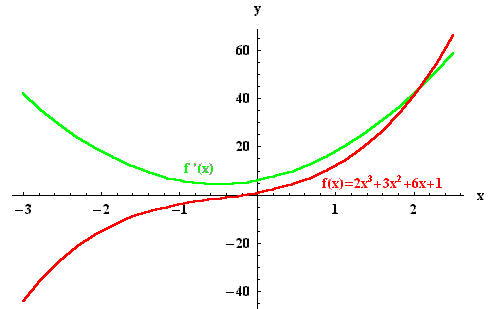What is the difference between the mean value theorem and the Rolle’s Theorem?

Mean value theorem
Rolle’s theorem

1.

Definition

Here function f is continuous on a closed interval [a, b] and differentiable on the open interval (a, b). Then there is at least one point c in (a, b) where
f '(c) = (f (b) - f (a)) / (b - a).

Here function f is continuous on a closed interval [a, b] and differentiable on the open interval (a, b). If f (a) = f (b), then there is at least one point c in (a, b) where f '(c) = 0.

2.

Tangent

At point c the tangent is parallel to the secant joining (a, f(a)) and (b, f(b))

At point c the tangent is parallel to the x-axis.

3.

Difference

It is the first mean value theorem. It is also known as Lagrange’s mean value theorem.

It is a special case of mean value theorem.

Mean Value Theorem
```### Course Features

• 731 Video Lectures
• Revision Notes
• Previous Year Papers
• Mind Map
• Study Planner
• NCERT Solutions
• Discussion Forum
• Test paper with Video Solution